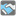• # Test doesnt run

## Question related to missionHow to Find Friends

I would like to give some feedback about ...

From: http://www.checkio.org/mission/find-friends/solve/

HTTP\_USER\_AGENT:

Mozilla/5.0 (Windows NT 6.1; WOW64) AppleWebKit/537.36 (KHTML, like Gecko) Chrome/36.0.1985.125 Safari/537.36

My Code:

def check_connection(network, first, second):
future = []
visited = []
dic = {}
for i in network:
a = i.split('-')
if a in dic and dic[a] != a:
dic[a].append(a)
else:
dic[a] = [a]
if a in dic and dic[a] != a:
dic[a].append(a)
else:
dic[a] = [a]
if [a] in dic.values():
for key,val in dic.items():
if a == key:
break
elif (''.join(ch for ch in val if ch.isalnum())) == str(a):
dic[str(a)].append(key)
break
if first in dic:
for i in dic[first]:
if i == second:
return True
else:
future.append(i)

while len(future) != 0:
for i in future:
for g in dic[i]:
if g == second:
return True
else:
future.append(g)
future.remove(i)
else:
return False